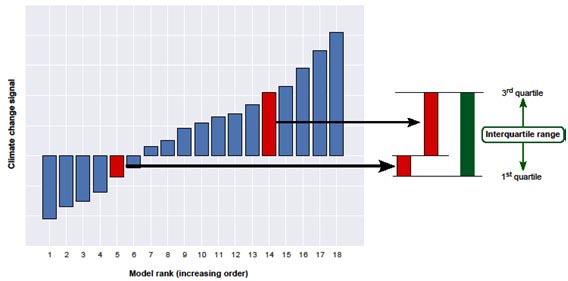## How to interpret ensemble value range?

The ensemble value range (interquartile range) is a confidence measure that provides information about the ensemble spread of the projected climate change signals. The ensemble value range indicates the range of the central half of the models, i.e. the models that group around the median of the models.

Figure 1 gives more details about the estimation of the ensemble value range. For a given grid point, all models are ordered according to their climate change signal. Then, the 1st and 3rd quartile model values are extracted (see box definition of quartiles). In our case, the 1st and 3rd quartiles correspond to the 5th (5th) and 14th (15th) model in the ranked sequence of 18 (19) models. The interquartile range is the difference between the 3rd and the 1st quartile.Figure 1 Schematic explanation of the interquartile range

Definition of the quartiles:

The 1st and 3rd quartiles are the 25th and 75th percentiles. If n is the total number of models, the 25th percentile, for e.g., corresponds to the model having the rank 0.25*n. In case the result is not an integer, it is rounded up. Example: n=18, 0.25*18=4.5, is rounded up to 5.

### Good practice in interpreting the ensemble value range

We recommend using the ensemble value range in combination with the confidence level
of agreement on sign of change and the ensemble mean change to retrieve some